@Team 2019-02-14T12:13:24.000000Z 字数 11105 阅读 2772

# 深入理解one-stage目标检测算法（上篇）

叶虎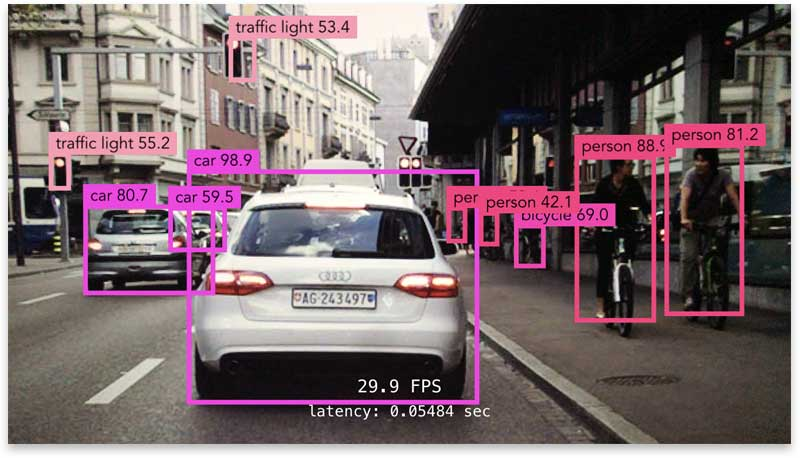## 为什么目标检测问题更难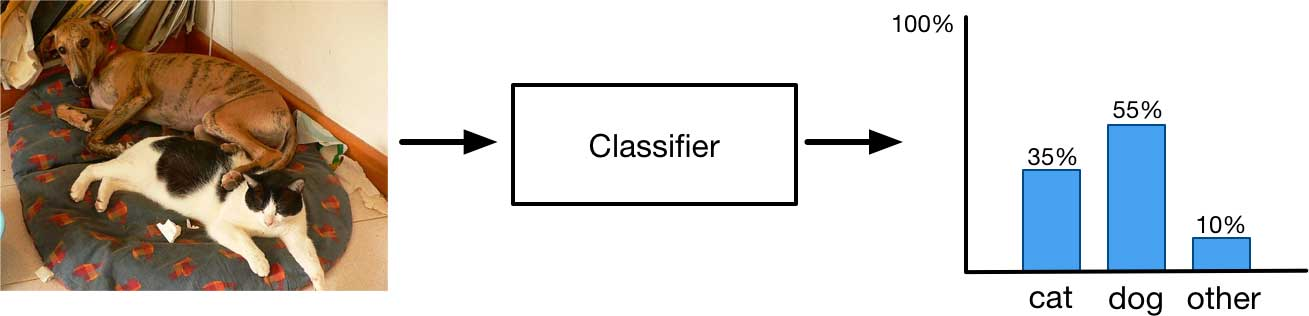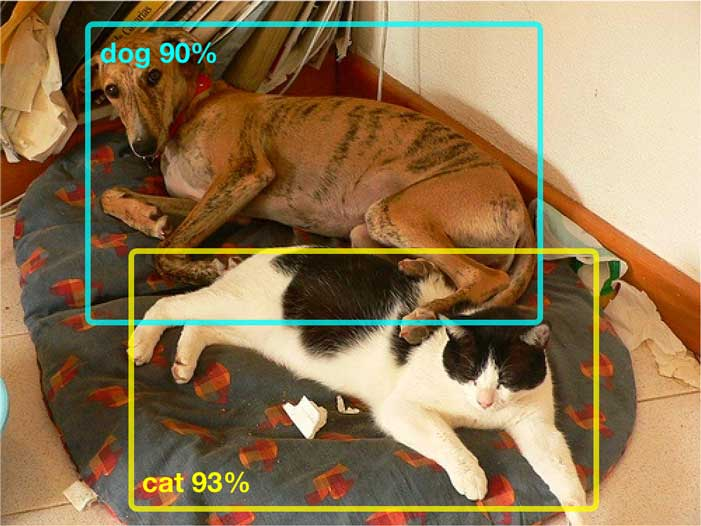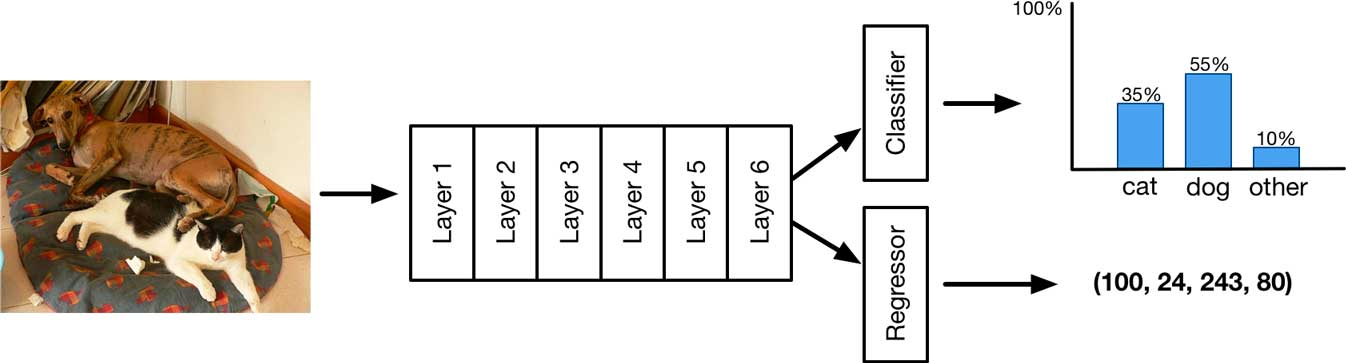outputs = model.forward_pass(image)class_pred = outputsbbox_pred = outputsclass_loss = cross_entropy_loss(class_pred, class_true)bbox_loss = mse_loss(bbox_pred, bbox_true)loss = class_loss + bbox_lossoptimize(loss)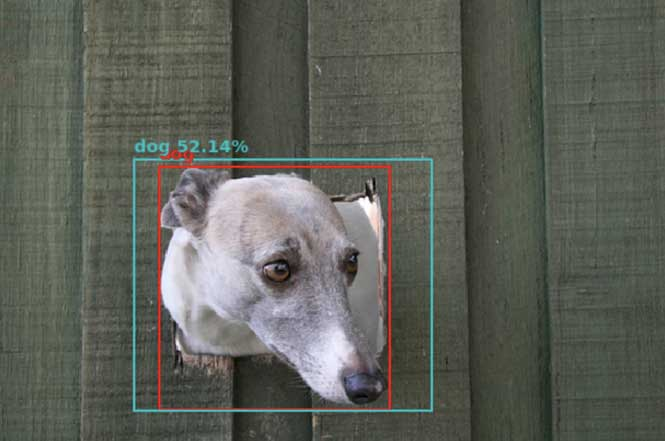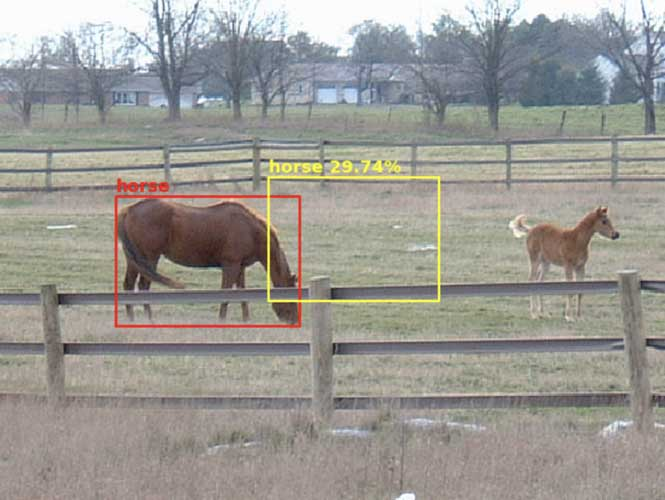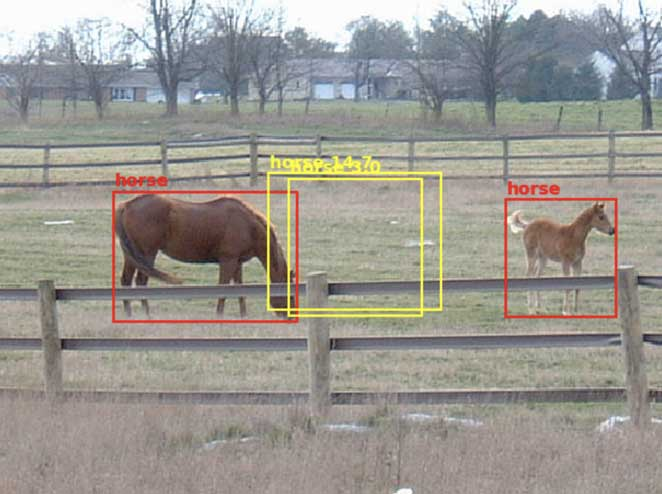## 使用网格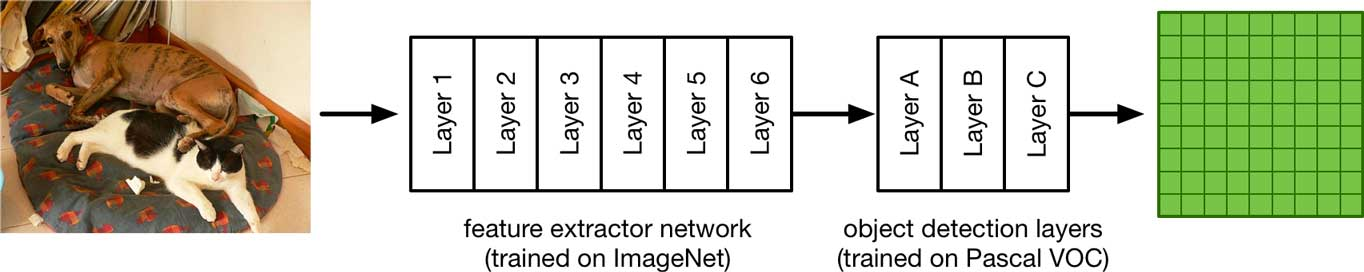YOLO或SSD的实际架构要比这个简单的示例网络稍微复杂一点，比如有残差结构，但我们稍后会介绍。目前，上述模型已经可用于构建快速且相当精确的目标检测模型。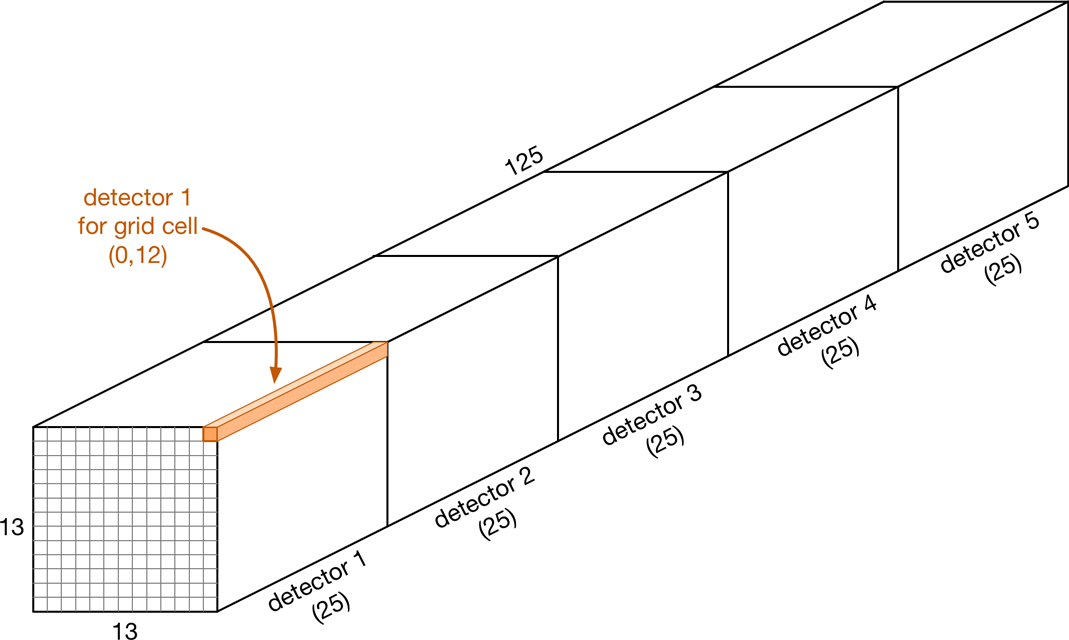• 表征类别概率的20个值
• 4个边界框坐标（中心x，中心y，宽度w，高度h）
• 1个值表示置信度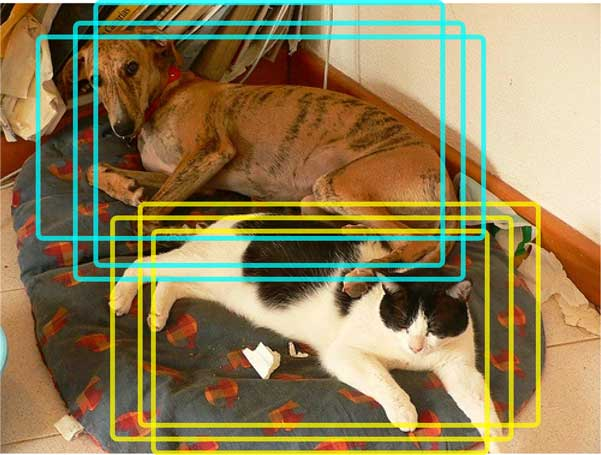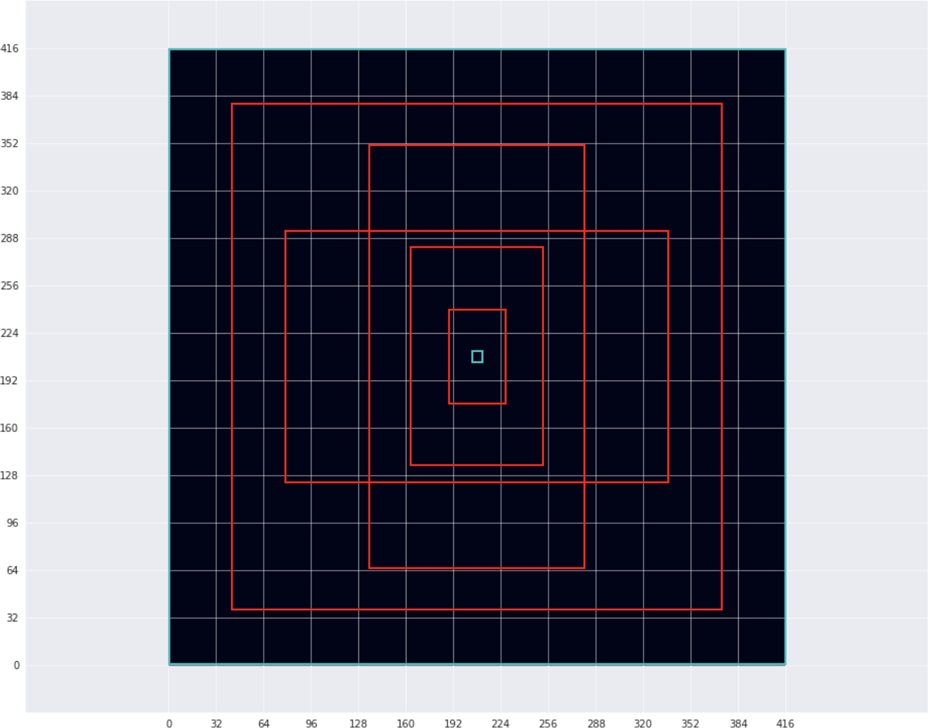anchors = [1.19, 1.99,     # width, height for anchor 1           2.79, 4.60,     # width, height for anchor 2           4.54, 8.93,     # etc.           8.06, 5.29,           10.33, 10.65]

YOLO通过在所有训练图像的所有边界框上进行k-means聚类来选择先验框（k=5，因此它找到五个最常见的物体形状）。因此，YOLO的先验框适合当前训练（和测试）的数据集。k-means算法中数据点是数据集中所有真实边界框的宽度和高度值。如果我们在Pascal VOC数据集的框中运行k-means，将得到5个簇：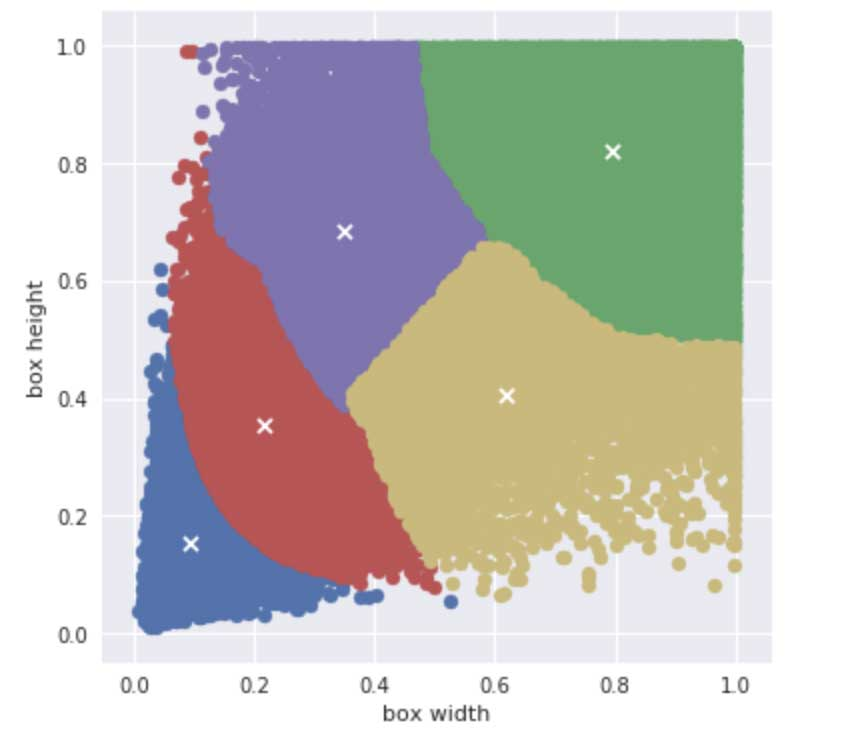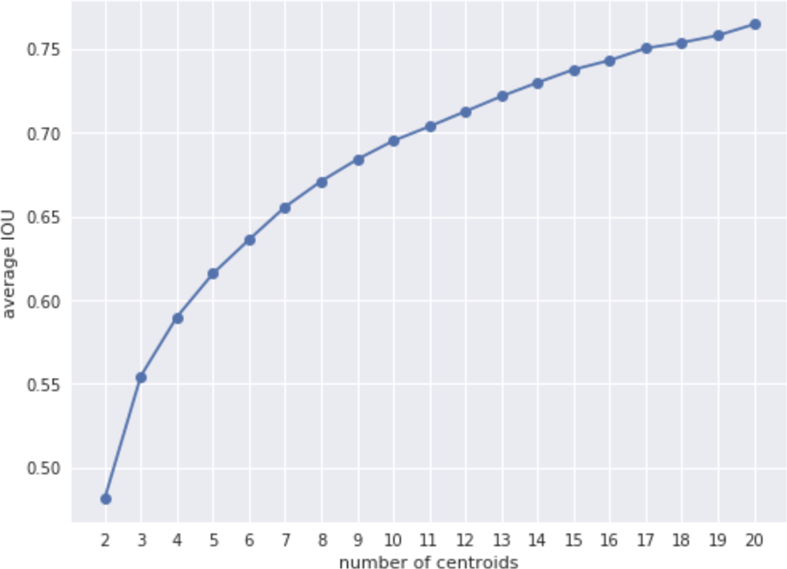SSD不使用k-means来确定先验框。相反，它使用数学公式来计算先验框尺寸，因此SSD的先验框与数据集无关（SSD称它们为“default boxes”）。SSD和YOLO的先验框设置，到底哪种方式比较好，并没有定论。另一个小差异是：YOLO的先验框只是宽度和高度，但SSD的先验框也有x，y位置。其实，YOLO也包含位置，只是默认先验框位置始终位于网格单元格的中心（对于SSD，先验框也是在网格中心）。

## 模型到底预测了什么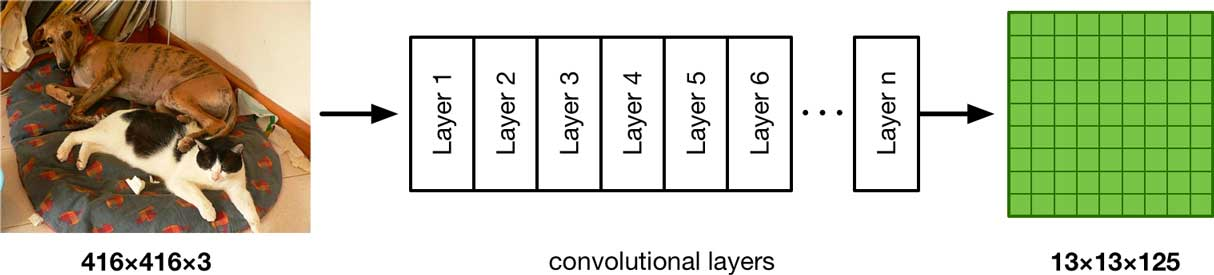• delta_x, delta_y：边界框中心点在网格单元内部的坐标；
• delta_w, delta_h： 边界框的宽和高相对先验框的缩放值。

box_w[i, j, b] = anchor_w[b] * exp(delta_w[i, j, b]) * 32box_h[i, j, b] = anchor_h[b] * exp(delta_h[i, j, b]) * 32

box_x[i, j, b] = (i + sigmoid(delta_x[i, j, b])) * 32box_y[i, j, b] = (j + sigmoid(delta_y[i, j, b])) * 32

YOLO模型的一个重要特点是仅当某个物体的中心点落在一个检测器的网格单元中心时，这个检测器才负责预测它。这样避免冲突，即相邻的单元格不会用来预测同一个物体。这样，delta_xdelta_y必须限制在0~1之间，代表预测框在网格单元的相对位置，这里用sigmod函数限制值的范围。然后我们加上单元格的坐标i和j（0~12之间），乘以每个单元格所包含的像素数（32）。这样box_xbox_y就表示预测框中心在原始416×416图像中的坐标。

box_x[i, j, b] = (anchor_x[b] + delta_x[i, j, b]*anchor_w[b]) * image_wbox_y[i, j, b] = (anchor_y[b] + delta_y[i, j, b]*anchor_h[b]) * image_h

confidence[i, j, b] = sigmoid(predicted_confidence[i, j, b])

classes[i, j, b] = softmax(predicted_classes[i, j, b])

confidence_in_class[i, j, b] = classes[i, j, b].max() * confidence[i, j, b]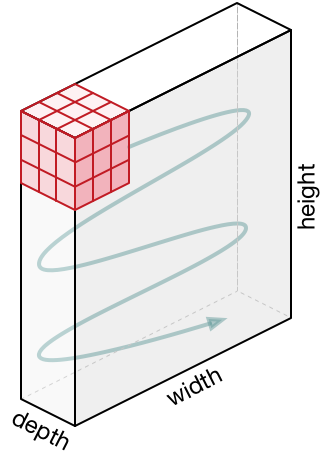## YOLO vs SSD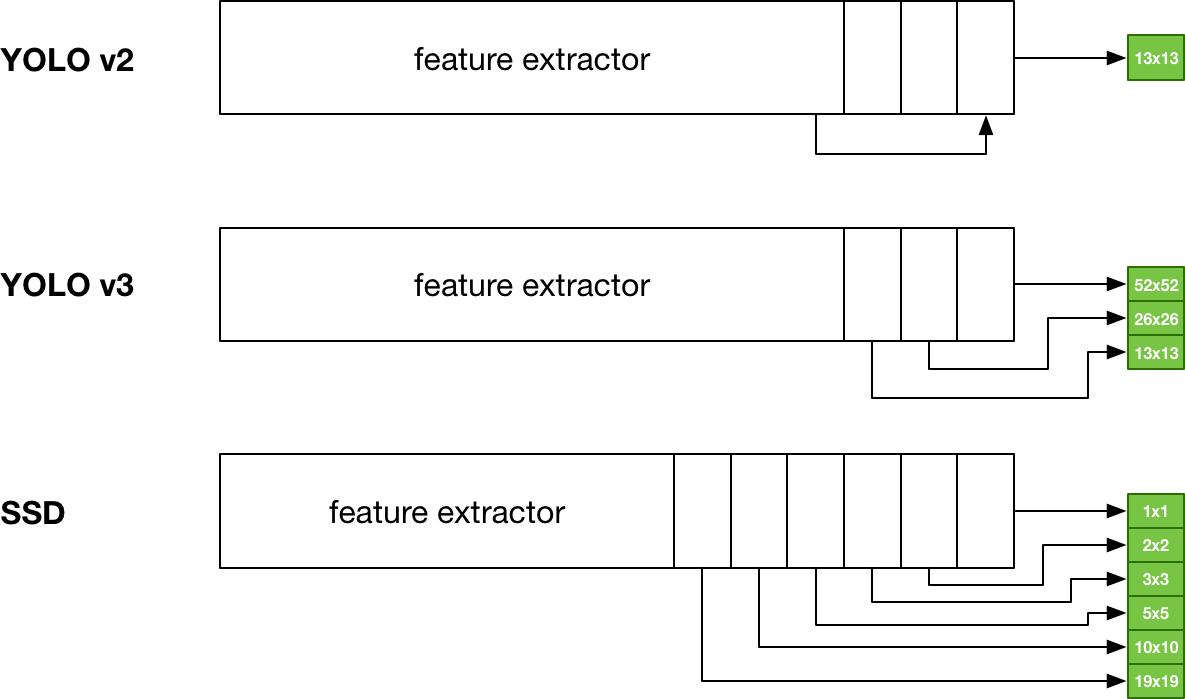SSD在先验框上与YOLO稍有不同。因为YOLO必须从单个网格进行所有预测，所以它使用的先验框的范围从小（大约单个网格单元的大小）到大（大约整个图像的大小）。SSD更为保守。19×19网格上的先验框比10×10网格上的要小，比5×5网格上的更小，以此类推。与YOLO不同的是，SSD不使用先验框来使检测器专注于物体大小，而是使用不同的网格来实现这一点。SSD的先验框主要用于使检测器处理物体形状（长宽比）的变化，而不是它们的大小。如前所述，SSD的先验框是使用公式计算的，而YOLO的先验框是通过对训练数据运行k-means聚类来找到的。• 私有
• 公开
• 删除